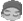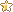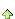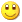共有937人关注过本帖树形打印复制链接

# 主题：利用Code128字体将文本转换为code128条形码ycs5801
1楼 | 信息 | 搜索 | 邮箱 | 主页 | UC加好友发短信搜遍了论坛也没找到之前有人做过这个。就是想把文本转换成code 128条形码，具体公式从网上找到的，求大神帮忙转换成易表的公式表示。Code128A```public string GetCode128A(string inputData) { string result = ""; int checksum = 103; int j = 1; for (int ii = 0; ii < inputData.Length; ii++) { if (inputData[ii] >= 32) { checksum += (inputData[ii] - 32) * (ii + 1); } else { checksum += (inputData[ii] + 64) * (ii + 1); } } checksum = checksum % 103; if (checksum < 95) { checksum += 32; } else { checksum += 100; } result = Convert.ToChar(203) + inputData.ToString() + Convert.ToChar(checksum) + Convert.ToChar(206); return result; }```ycs5801
2楼 | 信息 | 搜索 | 邮箱 | 主页 | UC加好友发短信Post By：2020/12/27 12:07:00 [只看该作者]

 Function StrToCode128(str As String) As String    Dim l As Integer, i As Integer, n As Integer, t As Integer, s As Integer    Dim p As String    l = Len(str)    n = 104    For i = 1 To l        t = Asc(Mid(str, i, 1))        If t >= 32 Then            n = n + (t - 32) * i        Else            n = n + (t + 64) * i        End If    Next i    s = n Mod 103    If s >= 95 Then        s = s + 100    Else        s = s + 32    End If    StrToCode128 = ChrW(204) + str + ChrW(s) + ChrW(206)   End Function这个vba版的